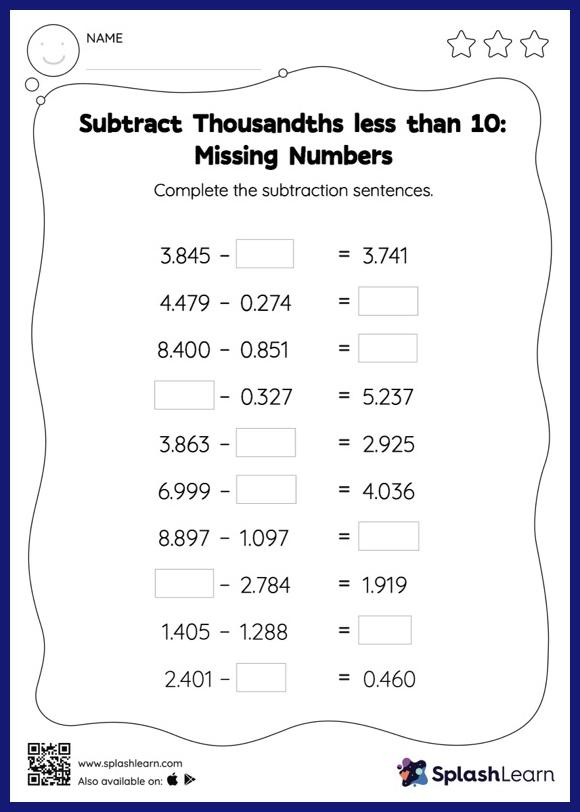# Subtract Thousandths less than 10: Missing Numbers Worksheet

Home > Subtract Thousandths less than 10: Missing NumbersThis worksheet consists of a set of questions on subtraction where students are required to subtract thousandths less than 10. When subtracting decimals on this worksheet, students align the decimal points and use zero as a placeholder. Then to find the missing number in the subtract thousandths less than 10 worksheet, students then apply the relationship between addition and subtraction.